# HBSE 10th Class Maths Solutions Chapter 11 Constructions Ex 11.2

Haryana State Board HBSE 10th Class Maths Solutions Chapter 11 Constructions Ex 11.2 Textbook Exercise Questions and Answers.

## Haryana Board 10th Class Maths Solutions Chapter 11 Constructions Ex 11.2

In each of the following, give also the justification of construction :

Question 1.
Draw a circle of radius 6 cm. From a point 10 cm away from its centre, construct the pair of tangents to the circle and measure their lengths.
Solution:
Steps of Construction :
1. Draw a circle with centre O of radius 6 cm.
2. Mark a point A, 10 cm away from the centre.
3. Join AO and bisect it at M.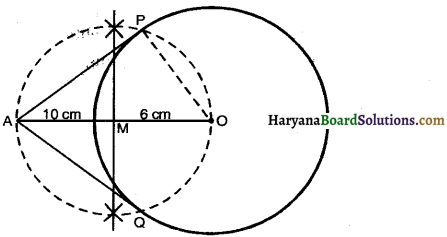4. Draw a circle with M as a centre and radius equal to AM intersects the given circle at points P and Q.
5. Join AP and AQ.
Then AP and AQ are the required tangents. Lengths of AP and AQ are 8-2 cm. (approx.)
Justification : Join OP
∠APO = 90° [angle in a semi-circle]
AP ⊥ OP
Therefore, AP is a tangent to the given circle.
Similarly AQ is a also tangent to the given circle.Question 2.
Construct a tangent to a circle of radius 4 cm from a point on the concentric circle of radius 6 cm and measure its length. Also verify the measurement by actual calculation.
Solution:
Steps of Construction :
1. O as the centre and draw two circles having radii 4 cm and 6 cm.
2. Taking point P on the larger circle.
3. Join OP and bisect it at M.
4. Taking M as the centre and radius equal to PM draw the circle which intersects the smaller circle at A and B.
5. Join PA and PB.
Then PA and PB are the required tangents.
On measuring the lengths of tangents
PA = PB = 4.47 (Approx.)
By calculation we have the length of tangent.
Joining AO
AO ⊥ PA [By theorem 10.1]
In right ∆PAO, we have
PO2 = PA2 + AO2
⇒ (6)2 = PA2 + (4)2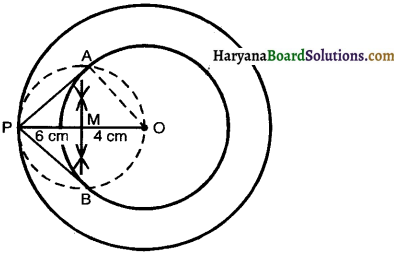PA2 = (6)2 – (4)2
PA2 = 36 – 16 = 20
⇒ PA = √20 = 4.472 cm
Justification : PA ⊥ OA
AO is the radius of the circle.
PA is tangent to a given circle.
Similarly PB is the tangent to the given circle.Question 3.
Draw a circle of radius 3 cm. Take two points P and Q on one of its extended diameter each at a distance of 7 cm from its centre. Draw tangents to the circle from these two points P and Q.
Solution:
Steps of Construction :
1. Draw a circle with centre O and radius equal to 3 cm.
2. Take points P and Q on extended diameter of the circle such that OP = OQ = 7 cm.
3. Mark mid point M1 of OP and mid point of OQ as M2.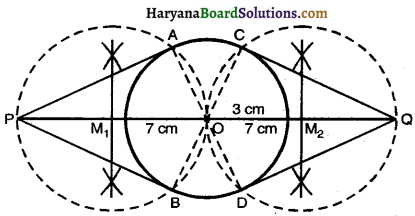4. Draw the circles as M1 and M2 centres and radii equal to PM1 and OM2 respectively, which intersects A and B (M1 as centre) and C, D (M2 as centre).
5. Join PA, PB and QC, QD.
Then PA, PB, QC and QD are tangents from the points P and Q respectively.
Justification : Join OA, OB, OC and OD ∠PAO = 90°
[Angle in a semi circle is 90°]
OA is the radius of circle.
PA is tangent to the given circle.
Similarly PB is the tangent to the given circle.
Again ∠OCQ = 90°
[Angle in a semi circle is 90°]
QC is the tangent to the given circle.
Similarly, QD is the tangent to the given circle.Question 4.
Draw a pair of tangents to a circle of radius 5 cm. which are inclined to each other at an angle of 60°.
Solution:
Steps of Construction :
1. Draw a circle with centre O and radius 5 cm.
2. Construct radii OA and OB such that ∠AOB = 360° – (90° + 90° + 60°) = 120°.
3. Draw AL ⊥ OA at A and BM ⊥ OB at B. They intersect at P.
Then PA and PB Eire the required tangents inclined to each other at 60°.
Justification : PA ⊥ OA and PB ⊥ OB and ∠AOB = 120°.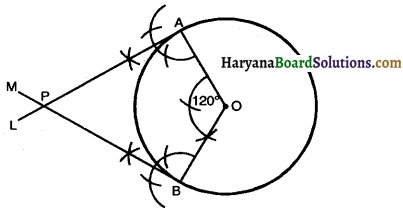⇒ ∠APB = 360° – 300°
⇒ ∠APB = 60°.
Therefore, tangents PA and PB are inclined at 60°.

Question 5.
Draw a line segment AB of length 8 cm. Taking A as centre, draw a circle of radius 4 cm and taking B as centre, draw another circle of radius 3 cm. Construct tangents to each circle from the centre of the other circle.
Solution:
Steps of Construction :
1. Draw a line segment AB = 8 cm.
2. A as centre and radius equal to 4 cm draw a circle.
3. B as centre and radius equal to 3 cm draw another circle.
4. Bisect line segment AB at M.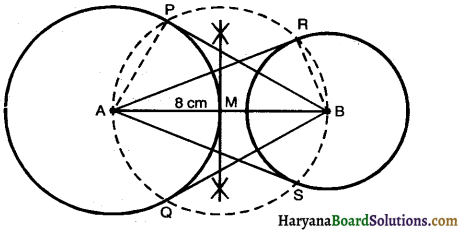5. M is the centre and radius equal to AM draw the circle which intersects the previous circles at P, Q (A as centre) and R, S (B as centre).
6. Join BP, BQ, AR and AS.
7. Then, BP, BQ, AR and AS are the required tangents.
Justification : Join AP, RB
∠APB = 90° [Angle in a semi circle is 90°]
PB ⊥ AP.
Therefore, AP is radius and BP is tangent.
Similarly, AR, AS and BQ are tangents.Question 6.
Let ABC be a right triangle in which AB = 6 cm, BC = 8 cm and ∠B = 90°. BD is the perpendicular from B on AC. The circle through B, C, D is drawn. Construct the tangents from A to this circle.
Solution:
Steps of Construction:
1. Construct ∆ABC such that BC = 8 cm, AB = 6 cm and ∠ABC = 90°.
2. Draw BD ⊥ AC.
3. ∠CDB = 90° So, BC is the diameter of circle passing through B, C, D.
4. Bisect line BC at O.
5. O as the centre, CO = OB as radius draw a circle which passes through B, C, D.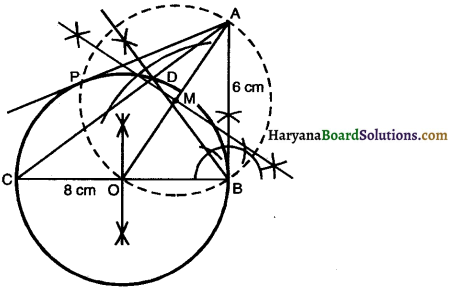6. Join AO which intersects the circle at M.
7. M as centre and MA = OM as radius draw another circle which intersect the previous circle at P and B.
8. Join PA.
Then, AP and AB are the required tangents.
Justification:
∠ABC = 90° [By Construction]
AB ⊥ OB.
OB is radius and AB is tangent.
Similarly, AP is tangent.Question 7.
Draw a circle with the help of a bangle. Take a point outside the circle. Construct the pair of tangents from this point to the circle.
Solution:
Steps of Construction :
Take four points A, B, C and D on the given circle and join AB and CD.
1. Draw the perpendicular bisectors of non parallel chords AB and CD which intersect at O.
2. O is the centre of circle.
3. Take a point P outside the circle.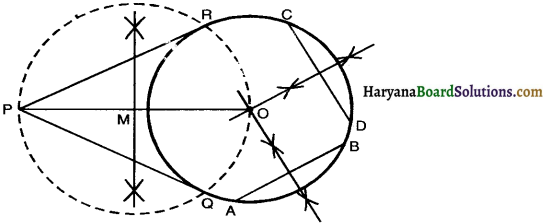4. Join OP and bisected it at M.
5. M as the centre and radius PM = MO, draw another circle which intersect previous circle at R.
6. Join PR and PQ.
Then PR and PQ are the required tangents.
Justification : Join OR,
∠PRO = 90° [Angle in a semi circle is 90°]
PR ⊥ OR
OR is a radius and PR is a tangent.
Similarly, PQ is a tangent.